# Parameter Passing by Reference

Large objects, or objects with expensive copy constructors, should not be passed by value because the creation of temporary copies consumes time, machine cycles, and memory needlessly. In C, we passed objects by pointer to avoid copying them. However, using pointers requires a different syntax from using regular variables. Further, accidental misuse of pointers can cause data corruption, leading to runtime errors that can be very difficult to find and fix. In C++ (and C99), we can pass by reference, which offers the same performance as a pointer-pass. With objects, this permits use of the (.) operator for accessing members.

A reference parameter is simply a parameter that is an alias for something else. To declare a parameter to be a reference, put the ampersand character (&) between the type name and the parameter name.

A reference parameter of a function is initialized by the actual argument being passed when the function is called. That argument must be, as with any reference, a non-const lvalue. Changes to a non-const reference parameter in the function cause changes to the actual object used to initialize the parameter. This feature is often exploited to allow functions, which can return at most one value, to cause changes in several objects, effectively allowing the function to return several values. Example 5.13 shows how reference parameters can be used with integers.

Example 5.13. src/reference/swap.cpp

 ```#include using namespace std; void swap(int &a, int &b) { int temp = a; cout << "Inside the swap() function: " << "address of a: " << &a << " address of b: " << &b << " address of temp: " << &temp << endl; a = b; b = temp; } int main() { int n1 = 25; int n2 = 38; int n3 = 71; int n4 = 82; cout << "Initial values: " << "address of n1: " << &n1 << " address of n2: " << &n2 << " value of n1: " << n1 << " value of n2: " << n2 << " address of n3: " << &n3 << " address of n4: " << &n4 << " value of n3: " << n3 << " value of n4: " << n4 << " Making the first call to swap()" << endl; swap(n1,n2); cout << "After the first call to swap(): " << "address of n1: " << &n1 << " address of n2: " << &n2 << " value of n1: " << n1 << " value of n2: " << n2 << " Making the second call to swap()" << endl; swap(n3,n4); cout << "After the second call to swap(): " << "address of n3: " << &n3 << " address of n4: " << &n4 << " value of n3: " << n3 << " value of n4: " << n4 << endl; return 0; } ```

There are extra output statements in Example 5.13 to help keep track of the addresses of the important variables.

```Initial values:
value of n1: 25 value of n2: 38
value of n3: 71 value of n4: 82
```

Initially our stack might look something like Figure 5.1:

Figure 5.1. Before first swap()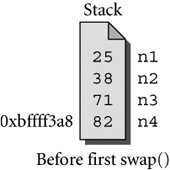As the program proceeds, we will see output like this:

```Making the first call to swap()
Inside the swap() function:
```

When references get passed to functions, the values that get pushed onto the stack are addresses, not values. Under the covers, pass-by-reference is very much like pass-by-pointer. Our stack now might look like Figure 5.2:

Figure 5.2. Inside first swap()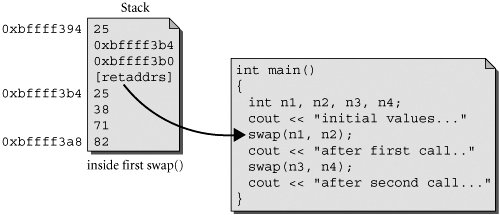```After the first call to swap():
value of n1: 38 value of n2: 25
Making the second call to swap()
Inside the swap() function:
```

Now our stack might look like Figure 5.3:

Figure 5.3. Inside second swap()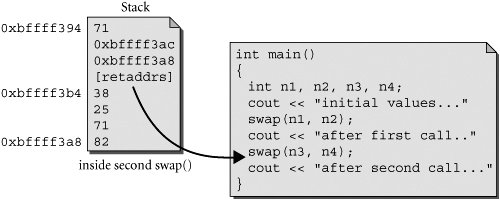```address of a: 0xbffff3ac address of b: 0xbffff3a8
After the second call to swap():
value of n3: 82 value of n4: 71
```

The swap() function is actually working with n1 and n2 during the first call and with n3 and n4 during the second call.

Pass-by-reference is an alternate syntax for passing by pointer. Under the covers, it is implemented the same way (i.e., the value is not copied). The main difference between pass-by-pointer and pass-by-reference is that you must de-reference a pointer, while a reference is accessible in the same way as the referred "thing."

Pass-by-Pointer or Pass-by-Reference?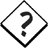When you have a choice, it is generally preferable to use references instead of pointers because this can reduce the number of places where a programmer can accidentally corrupt memory. It is only when you need to manage objects (creation, destruction, adding to a managed container) that you need to operate on pointers, and those routines can usually be encapsulated as member functions.

### References to const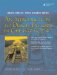An Introduction to Design Patterns in C++ with Qt 4
ISBN: 0131879057
EAN: 2147483647
Year: 2004
Pages: 268